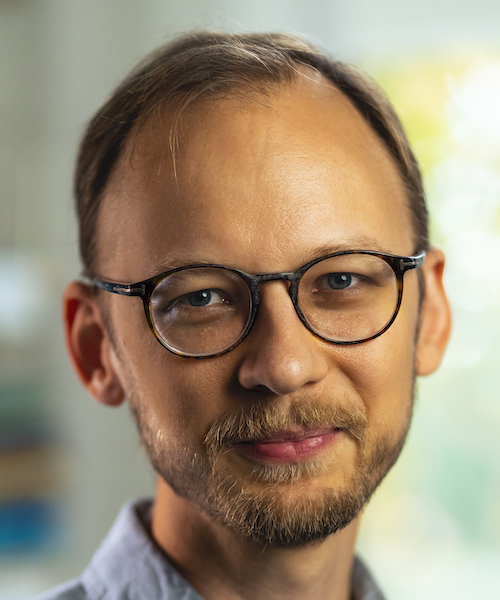Professor at KTH (Algebraic Geometry) Office: 3546 Phone: +46-8-790-7179 Address Department of Mathematics Royal Institute of Technology (KTH) SE-100 44 Stockholm Sweden E-mail: dary AT math.[university-name].se Algebraic geometry group at KTH Algebraic topology group at KTH Algebra and geometry seminars at KTH Algebra, geometry, topology and combinatorics at SU
 Current activities Mittag-Leffler program: Moduli and algebraic cycles, Fall 2021 Workshop: Motives and Hodge theory, Oct 18–22, 2021 Workshop: Moduli spaces and log geometry, Nov 15–19, 2021
 Research interests Algebraic and arithmetic geometry: Algebraic stacks and spaces, moduli problems. Birational geometry (flatification, compactification, weak factorization). Blow-ups of various kinds: stacky, weighted, derived, saturated, ... (Non-reductive) geometric invariant theory. Derived algebraic geometry. Derived and triangulated categories, monoidal categories, coherent functors. Resolution of singularities. Wild ramification, Riemann—Zariski spaces, non-archimedean geometry, valuation theory, log geometry. Motives, motivic homotopy theory. Chow and Hilbert schemes, Moduli of higher-dimensional varieties. Divided powers, seminormality, tight closure. CV